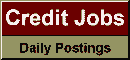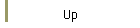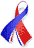DefaultRisk.com the web's biggest credit risk modeling resource.doi> search: A or B Export citation to:- HTML- Text (plain)- BibTeX- RIS- ReDIF

A Model of Bankruptcy Prediction

by Eivind Bernhardsen of the Norges Bank

December 5, 2001

Abstract: In this thesis, a model of bankruptcy prediction conditional on financial statements is presented. Apart from giving a discussion on the suggested variables the issue of functional form is raised. The specification most commonly applied for the bankruptcy prediction model implies that the rate at which two variables can substitute another holding predicted risk unchanged will be constant. If the aspect captured by single financial ratios is considered less a substitute for any other aspect as this ratio grows, this restriction may not be appropriate. Specifically, the structure of constant compensation will make predictions sensitive to non-credible outliers. A specification of the logit model which allows for flexible rates of compensation is motivated. The model is estimated and the regression results are reported. Second; by questioning the direct connections between financial ratios and the particular outcome of bankruptcy, a model structure which determines an upper bound on probability estimates is explored. By reference to a simple model of misclassification, the specification distinguishes between the probability of bankruptcy and the probability of insolvency. Whereas the predicted probabilities of bankruptcy can be evaluated empirically, the event of insolvency is not observable. Nevertheless; conditional on the model structure, probabilities can be derived for this event as well. An evaluation is given on the ability of the model to measure the over-all development in credit risk for the Norwegian limited liability sector. Individual probabilities of bankruptcy are multiplied with the firms debt to generate a prediction of expected loss in absence of recovered values. This measure is then aggregated and fitted with total loan losses for the Norwegian banking sector over the years 1989-2000. Finally, the possibility of assessing the effect of macro variables in a short panel of firms is explored. With reference to an aggregation property of the probit model, a suggestion is given on how to estimate time-specific effects on aggregate data as a means to identify macro coefficients that can be included in the micro-level model.

Keywords: Bankruptcy, logit analysis, non-linear estimation, aggregation.

Books Referenced in this paper:  (what is this?)